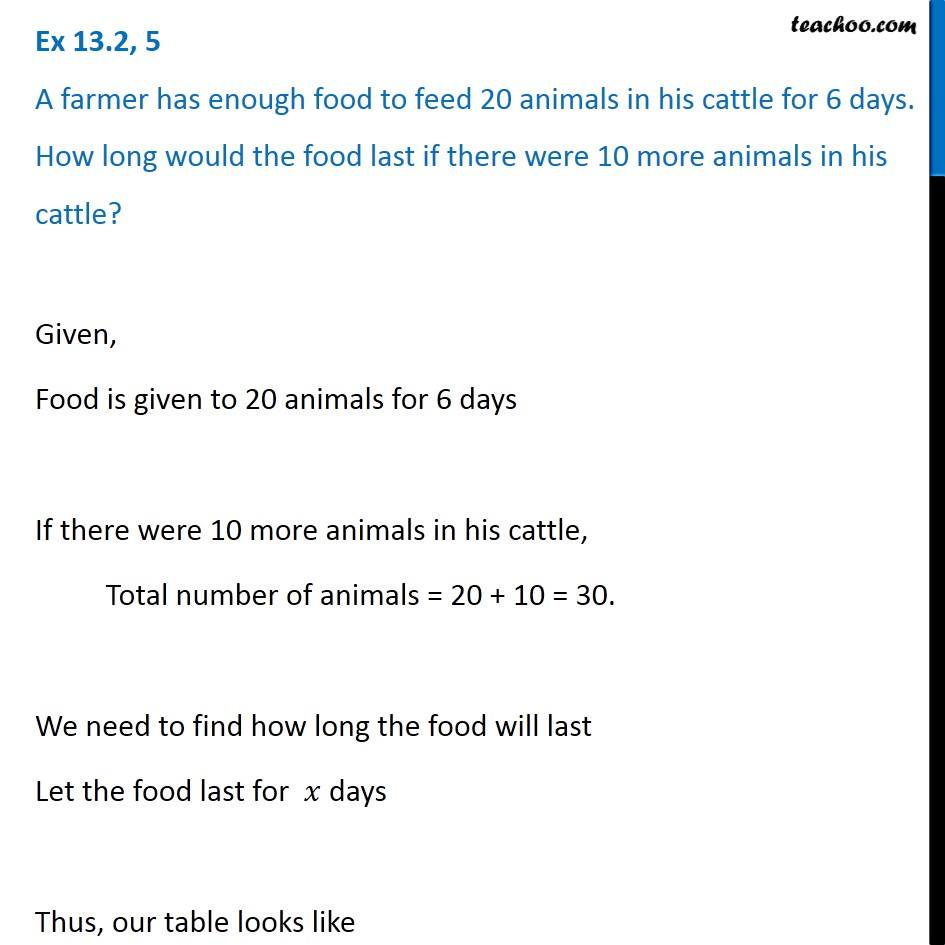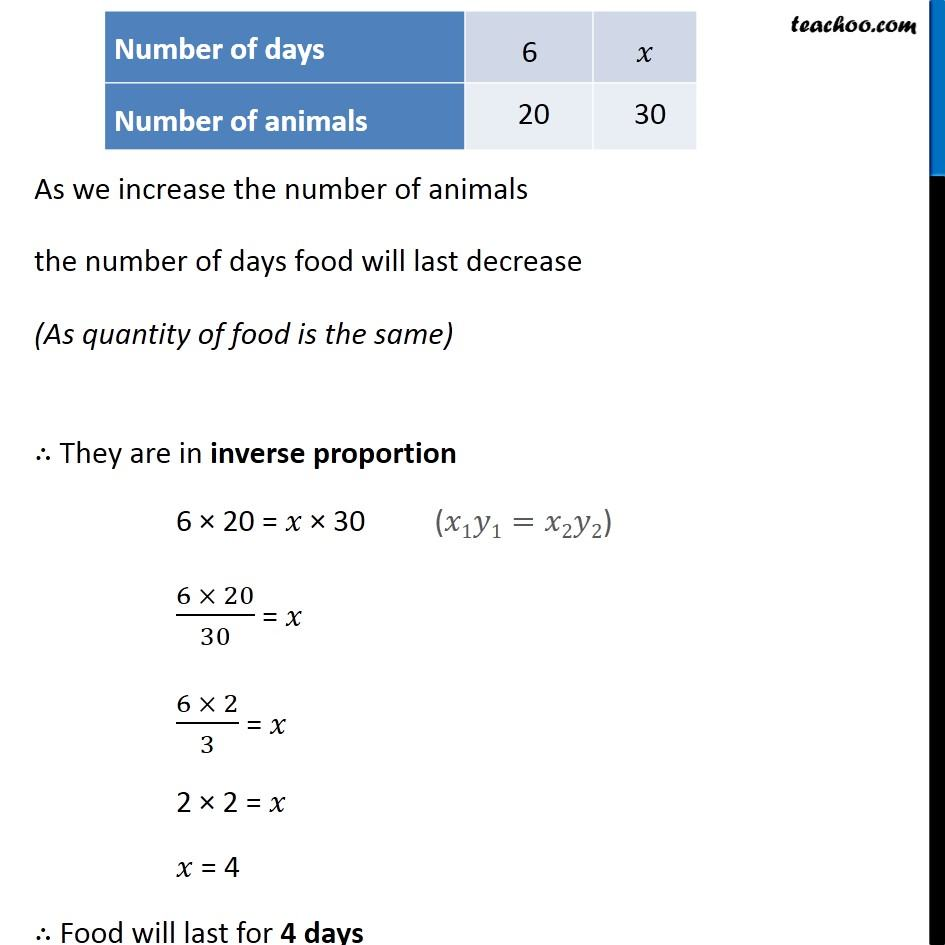1. Chapter 13 Class 8 Direct and Inverse Proportions
2. Concept wise
3. Inverse Proportion

Transcript

Ex 13.2, 5 A farmer has enough food to feed 20 animals in his cattle for 6 days. How long would the food last if there were 10 more animals in his cattle? Given, Food is given to 20 animals for 6 days If there were 10 more animals in his cattle, Total number of animals = 20 + 10 = 30. We need to find how long the food will last Let the food last for 𝑥 days Thus, our table looks like As we increase the number of animals the number of days food will last decrease (As quantity of food is the same) ∴ They are in inverse proportion 6 × 20 = 𝑥 × 30 (6 × 20)/30 = 𝑥 (6 × 2)/3 = 𝑥 2 × 2 = 𝑥 𝑥 = 4 ∴ Food will last for 4 days

Inverse Proportion